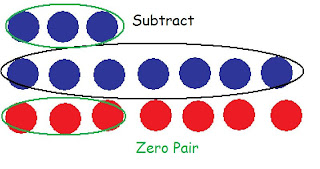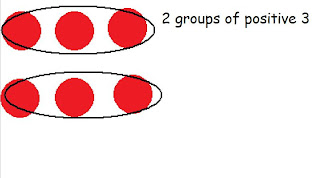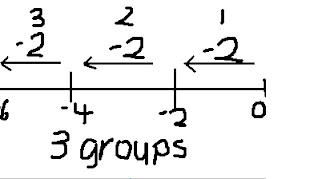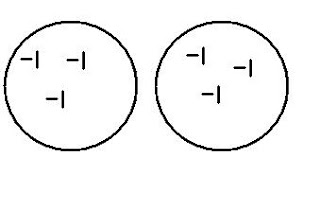## Tuesday, March 22, 2011

### Errol's Great Big Book of Integers

-A zero pair are 2 integers that have the same value but has opposite signs.
Ex. (+9) and (-9) equal 0
-Integer problems can be solved using a number line or chips. When using chips a red chip would represent a positive integer and a blue chip would represent a negative integer, so one blue chip and one red chip would equal a zero pair.
-A rule to remember when subtracting integers is "when subtracting something that isn't there use a zero pair"
In class we where asked to solve these problems:
1) -3 - (-7)= +42) -3 -7= -103) 3 -7= -44) 3 + 7= 105) -3 +7Chapter 2: Multiplying Integers

Sign rule: when you have an odd amount of negative signs the product will be negative, when you have an even amount of negative signs the product will be positive. Here are some examples:
1. (+2) x (+3)= 6(+2) x (-3)= -6
(-2) x (+3)= -6
(-2) x (-3)= +6

How I solved these questions:

Chapter 3: Dividing Integers

Sign Rule Works with Dividing Integers too. When you have an odd amount of negative signs the quotient will be negative, when you have an even amount of negative signs the quotient will be positive.

Partitive Division is how many groups are between 0 and a number.
Example 6÷2=3-6÷ (-2)=3Quotative Division is
when finding how many
objects are in each group then finding how many
groups there are.

How do you share (-6) with 2 groups?
(-6)÷2= -3Multiplicative Inverse is when you switch the quotient with the divisor or dividend.
Example: 6÷(-2)=
Make the question: -2 ÷ _ = 6. So 2 x 3 is 6 and since the 2 is negative, the 3 has to be negative because the answer is positive.
6÷2= answer would be positive because there are no negatives
-6÷ (-2)= answer would be positive because there is an even amount of negatives which is 2
(-6)÷2= answer would be negative because there is an odd amount of negatives which is 1
6÷(-2)= answer would be negative because there is an odd amount of negatives which is 1

Chapter 4:
Order of Operations with Integers
BEDMAS is an order of operation
B- Brackets
E- Exponents
D- Division
M- Multiplication
S- Subtraction

(+5) x (-3) + (-6) ÷ (+3)= First we look for bracketed operations, since there are
none we look for multiplication or division looking from
left to right, and since there are I put a square bracket.

[(+5) x (-3)] + (-6) ÷ (+3)= Now we do the multiplication in the square brackets.

(-15) + (-6) ÷ (+3)= Now we square bracket the division.

(-15) + [(-6) ÷ (+3)]= Now we do the division in the square bracket.

(-15) + (-2)= Now add the numbers.

= (-17)